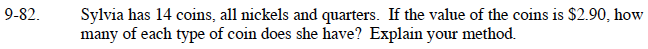### Home > GC > Chapter 9 > Lesson 9.2.3 > Problem9-82

9-82.Write a system of equations where n represents number of nickels and q is number of quarters.

n + q = 14

0.05n + 0.25q = 2.90

Use the substitution method explained in the Math Notes box in Lesson 2.1.3 to solve for x.

n = 3
q = 11